# 2-sphere in Euclidean space

(diff) ← Older revision | Latest revision (diff) | Newer revision → (diff)
Arbitrary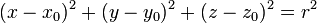$(x - x_0)^2 + (y - y_0)^2 + (z - z_0)^2 = r^2$$(x_0,y_0,z_0)$ are the coordinates of the center and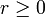$r \ge 0$ is the radius of the sphere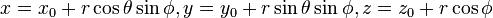$x = x_0 + r \cos \theta \sin \phi, y = y_0 + r \sin \theta \sin \phi, z = z_0 + r \cos \phi$$\theta$ and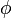$\phi$ play roles analogous to the azimuthal and polar angles. See spherical polar coordinates.
Up to translations, i.e., given any 2-sphere in Euclidean space, we can do a translation and bring it into this form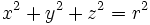$x^2 + y^2 + z^2 = r^2$$r \ge 0$ is the radius.$x = r \cos \theta \sin \phi, y = r \sin \theta \sin \phi, z = r \cos \phi$.$\theta$ and$\phi$ play roles analogous to the azimuthal and polar angles. See spherical polar coordinates. We have used a translation to move the center of the sphere to the origin.
Up to all rigid motions (translations, rotations, reflections)$x^2 + y^2 + z^2 = r^2$$r \ge 0$ is the radius. We have used a translation to move the center of the sphere to the origin.$x = r \cos \theta \sin \phi, y = r \sin \theta \sin \phi, z = r \cos \phi$.$\theta$ and$\phi$ play roles analogous to the azimuthal and polar angles. See spherical polar coordinates. Since the sphere has rotational and reflection symmetry, allowing freedom of rotation does not result in any simplification of the equation.
Up to all similarity transformations (transformations, rotations, reflections, scaling)$x^2 + y^2 + z^2 = 1$ no parameters any more. This is the unit 2-sphere centered at the origin.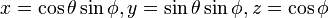$x = \cos \theta \sin \phi, y = \sin \theta \sin \phi, z = \cos \phi$.$\theta$ and$\phi$ play roles analogous to the azimuthal and polar angles. See spherical polar coordinates. We've scaled the sphere to unit radius.